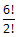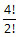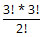# TANCET 2013 Quant Qn 46: Permutation

## Question

In how many ways can the letters of the word BEAUTY be rearranged such that the vowels always appear together?

1.2. 4! * 3!
3.4.5.Correct Answer    Choice 2. 4! * 3!

• ### Rearranging Objects - Concept

Let us quickly understand the number of ways objects can be rearranged.

Let us take 3 objects A, B, and C and arrange them in 3 places 1, 2, and 3.

Start by placing one of the objects, say A.
A can be placed in any of the 3 places viz., 1, 2 or 3. So, A has 3 choices.
Let us place A in the 2nd place.

Now, let us place the second object, say B.
B can be placed only in one of the two vacant slots viz., 1 or 3. So, B has 2 choices.
Let us place B in the 3rd place.

The last object C can be placed in the only remaining place in only 1 way.

Here is the computation to find the number of ways to rearrange these 3 objects

A can be placed in a slot in 3 ways and B can be placed in a slot in 2 ways and the C can be placed in a slot in 1 way.
Therefore, the total number of ways of placing the 3 objects = 3 * 2 * 1 = 3! (factorial 3) = 6 ways.

The table given below lists all 6 ways of rearranging 3 objects in the 3 slots.

Slot 1 Slot 2 Slot 3
A B C
A C B
B A C
B C A
C A B
C B A

If we have 4 objects A, B, C and D, these 4 objects can be rearranged in 4 * 3 * 2 * 1 = 4! ways.
We can generalize the above result as follows: r objects, if they are all distinct, can be rearranged in r! ways.

• ### Solution to the question

BEAUTY is a 6-letter word.

The vowels among the 6 letters are E,A, and U.

The question stipulates that the vowels should appear together. i.e., EAU should appear as 1 unit.

Let us call this unit X.

So, we need to re arrange BXTY.

4 distinct objects can be rearranged in 4! ways.

We have one more step to cover before arriving at the final answer.
The unit of vowels, EAU (3 distinct letters, which we have taken as X) can be rearranged in 3! ways.

Therefore, the total number of ways of reordering BEAUTY such that the vowels appear together is 4! * 3!

The correct answer is Choice 2. 4! * 3! ways.

## Online TANCET MBA CourseTry it Free!

Register in 2 easy steps and start learning in 5 minutes!